RUSSIAN JOURNAL OF EARTH SCIENCES, VOL. 18, ES3003, doi:10.2205/2018ES000623, 2018

# On the monitoring of seismic activity using the algorithms of discrete mathematical analysis

B. A. Dzeboev1,2, R. I. Krasnoperov1

1Geophysical Center of the Russian Academy of Sciences, Moscow, Russia

### Abstract

The paper presents a new mathematical approach, entitled Seismic Activity monitoRing by Discrete mathematical analysis (SARD) and aimed at seismic level assessment. It is based on application of well-proven algorithms of Discrete Mathematical Analysis (DMA) for the study of earthquake catalogs. The possibility of applying the proposed method for the territory of California, Kamchatka Peninsula and the Caucasus is shown. Evaluation of efficiency of the developed method is carried out using an error diagram.

### Introduction

The problem of reliable estimation of seismic hazard is an important task not only for seismology, but for all geophysical studies. Its solution is of great social and economic importance. The urgency of the problem rapidly increases with the vigorous growth of urban territories. According to the UN statistics, seismic catastrophes account for more than 50% of the total number of natural disasters and dominate all types of catastrophes. Earthquakes are the most complex natural phenomena associated with the dynamics of lithospheric plates, the accumulation of tectonic stresses and their drops. Strongest earthquakes lead to serious economic and financial loss and numerous casualties. A significant part of the territory of the Russian Federation (about 25%), which resides 27 Russian regions with a population of more than 20 million people, is situated in seismic zones. Here, recreational facilities are developing rapidly.

Over the past 35 years in many countries, which are characterized by significant seismic activity, including occurrence of strongest earthquakes, scientific research on the creation of methods for earthquake prediction has been significantly developed. The experience amassed in international geophysics testifies that the creation of systems of complex observations, providing for the required sampling rate in space and time, a continuous collection of prognostic data with the leading role of seismological observations, is of fundamental importance for the successful solution of the problem of determining the place, time and strength of the expected earthquake [Laverov et al., 2008]. The countries that have reached the highest level of methods and techniques for seismic risk assessment and earthquake prediction are Japan, the USA and China. China has the most powerful system of seismological monitoring, oriented on earthquake prediction. Several thousand seismic stations operate in the USA. In most earthquake-prone areas, for example in California, the density of the seismic network is such that the average distance between stations is about 10 km. Seismological monitoring of the territory of USA is carried out by the US Geological Survey (http://www.usgs.gov/). High density of networks of seismological and geophysical observations is typical for the territory of Japan. However, in comparison to China, research on earthquake prediction in Japan is coordinated to a much lesser extent.

The state of seismological observations in Russia is characterized by departmental disunity of the conducted work, decrease in the level of financial support of observational networks, and technical deterioration of equipment [Laverov et al., 2008]. Currently there are no sufficiently developed observational networks for monitoring within seismically active regions of Russia. All these factors significantly reduce the provision of seismic safety for the population over the territory of this country. In this regard, the development of a mathematical approach for monitoring the rate of seismic regime in the regions of Russia on the basis of discrete mathematical analysis (DMA) is certainly topical.

To predict an earthquake means to determine with sufficient reliability its location, time and magnitude. Hence, it is necessary to know how and where the earthquake source is being prepared and to monitor the successive stages of its development.

The physical and geological basis and the principal possibility of forecasting earthquakes are determined by two conditions. First, the strength of the rocks composing the Earth's crust is heterogeneous: the stronger sections alternate with the weak ones. Secondly, the stresses accumulate slowly, for hundreds of years. Under these conditions, the preparation of a large rupture is preceded by successive acts of destruction of many less durable sites, each of which causes a small earthquake. Observing the weak seismicity, it is possible to identify the place and estimate the strength of a future strong earthquake.

Typically, medium-term precursors appear several years or months before the main strong earthquake [Novikova and Rotvain, 1996]. They are based on the following characteristics of the seismic flow: the level of seismic activity, its variations over time, and the spatiotemporal grouping of earthquakes [Keilis-Borok et al., 2001].

A new approach to the analysis of geophysical data (discrete mathematical analysis, DMA), created and developed at the Geophysical Center of the Russian Academy of Sciences, is based on fuzzy mathematics and provides efficient monitoring of dynamic geophysical processes irregular in time and space, highlighting the background or normal component of the behavior of these processes and the anomalous component. At the same time, during such monitoring, the researcher himself determines the "virtual" nodes of the grid. They may not coincide with the nodes of real observations and there may be more of them than nodes of real observations.

DMA makes it possible to monitor the time- and space-dependent finite dynamic process. The essence of monitoring includes digitization, topological filtration, association with monitoring nodes, the aggregation of time series, and finally recognition of quiet/anomalous structures.

### DMA-Monitoring of Seismic Level

Discrete mathematical analysis is a new approach to data analysis, developed at the Geophysical Center of the Russian Academy of Sciences. DMA is a series of algorithms aimed at solving the main tasks of data analysis: clustering and tracing in multidimensional arrays [Agayan et al., 2014; Agayan and Soloviev, 2004; Gvishiani et al., 2017b, 2017c; Mikhailov et al., 2003; Soloviev et al., 2016; etc.], morphological analysis of surface [Gvishiani et al., 1994, 2008d; etc.], search for anomalies and trends in records [Gvishiani et al., 2003, 2004, 2008a, 2008b, 2008c; Soloviev et al., 2012a, 2012b; etc.]. All DMA algorithms are united by a common formal basis, based on fuzzy comparisons of numbers and proximity measures in discrete spaces. The idea of DMA is to create discrete analogues of the concepts of classical mathematical analysis: limit, continuity, smoothness, connectivity, monotonicity, extremum etc. DMA algorithms and their combinations provide a way to monitor the seismic process, irregular both in time and in space.

Let's consider an example: $X$ is any seismic catalog in the domain $\Omega$. The essence of monitoring:

0. The beginning is digitization. In time it is division into equal intervals $\{\delta t_i\}$. In space it is selection of monitoring nodes $\{\omega_j\}$ in the domain $\Omega$.

1. Clustering (topological filtration – allocation of a reference dense subset $Y_i$ in the image $X(\delta t_i$) of the process $X$ in the interval $\delta t_i$ by means of the algorithm discrete perfect sets (DPS) [Gvishiani et al., 2013a, 2013b, 2013c]: $Y_i= \mathrm{DPS}(X(\delta t_i$)).

2. Transfer of clustering to the monitoring nodes (the spatial grid of interest) is the construction of the fuzzy activity measure $\mu (\omega_j) (i) \in [0, 1]$ [Soloviev et al., 2013] by the DMA methods for each node $\omega_j$ relative to the process $X$ for the period $\delta t_i$ through the proximity $\omega_j$ to $Y_i$.

3. Postulation – monitoring Mon$(X)$ is the aggregation of time series in the monitoring nodes $\{ \mu(\omega_j)(i)\}$.

4. Statistical, wavelet and DMA-analysis Mon$(X)$ with subsequent conclusions on the grid $\omega_j$. In particular, recognition of quiet/anomalous structures within a grid.

SARD (Seismic Activity monitoRing by Discrete mathematical analysis) [Dzeboev, 2017], a method we apply here, continues a series of the DMA methods successfully applied to the analysis of earthquake catalogs. The seismic process is analyzed by studying its behavior at the nodes of the coordinate grid with a given interval (reference points) and constructing measures of activity. As a measure of activity, we use the value $\mu$, determined by the algorithm of topological filtration (clustering) DPS [Agayan et al., 2014; Gvishiani and Dzeboev, 2015; Gvishiani et al., 2013a, 2013b, 2016, 2017c] or its adaptive version A-DPS (adaptive discrete perfect set).

The DPS algorithm is the part of DMA [Gvishiani et al., 2008a], an algorithmic approach that is being developed in the Geophysical Center of RAS under the leadership of A. D. Gvishiani. It is included in its DMA-clustering block [Agayan and Soloviev, 2004; Gvishiani et al., 2008d]. DMA-clustering algorithms solve the problems of topological filtering of multidimensional data sets, highlighting in these arrays the most significant parts and cutting off the non-essential ones. DPS is the result of further development of ideas presented in [Agayan et al., 2014]. As the results of the research showed [Gvishiani et al., 2013a, 2016; etc.], it can be used for recognizing the locations of strong earthquakes' possible occurrence along with the classical EPA method (Earthquake-Prone Areas recognition) [Gvishiani and Dubois, 2002, Gvishiani et al., 2017a; Soloviev et al., 2014].

The calculated measure $\mu$ varies within the interval $[-1, 1].$ In contrast to the classical measure of seismic activity [Riznichenko, 1967], the measure $\mu$ reflects the relative density of epicenters that varies over time in comparison with the spatial environment. A local increase in time of the parameter $\mu$ may reflect an increase in the activity of weak earthquakes, often accompanying the final stage of the preparation of a strong earthquake [Keilis-Borok et al., 2001; Kossobokov and Shebalin, 2003]. Thus, the variation of $\mu$ in time can be also used for diagnosis of periods of seismic hazard level increase and for estimating the forthcoming earthquake location.

By the monitoring of seismic activity, we mean the analysis and study of the behavior of the set of time series for measure $\mu$ at the reference points. Time series are constructed with a constant step. At each step, the time interval $t_i$ is considered. For each interval, with the use of the DPS or A-DPS algorithm, a dense subset of epicenters of earthquakes (clusters) is allocated. The idea of application of the DPS algorithm ($\beta, q$) for solving the task of seismic activity monitoring is to use its free input parameter $\beta$ – the level of the maximum density of the determined dense clusters of epicenters of earthquakes (we used the input parameter $q$ to calculate the localization radius). Parameter $\beta \in [-1, 1]$ allows the results of the DPS analysis of the earthquake catalog for a certain period of time $t_i$ to be transferred to the nodes of the regular geographic grid covering the investigated region by constructing for each node the measure $\mu (t_i)$ of the activity of the seismic process in its vicinity for the period of time $t_i$. For this purpose, the earthquake catalog is preliminarily divided into time intervals $t_i$. For each $t_i$, a DPS clustering of the earthquake catalog with discretely varying values of parameter $\beta$ is carried out and a measure $\mu (t_i$) corresponding to the maximum parameter $\beta^* \in [-1, 1]$ is assigned to each grid node, at which the node is close to the distinguished DPS$(\beta^*, q)$-clusters of earthquake epicenters. If the node is not close to any of the subsets selected by the DPS algorithm, then its measure of activity is $-1$.

Since the seismic process in a certain spatial neighborhood is irregular in time, and the change in the level of its activity, often indicating an increase or decrease in potential seismic risk, occurs over a certain time interval, when assessing seismic activity at time $t_i$, it is necessary to take into account its time memory. For this, at each instant of time $t_i$, the value of measure $\mu$ is recalculated with respect to the memory in the time interval $[t_{i-\Delta}, t_{i-1}]$ in the form of power averaging with weighting coefficients:

\begin{eqnarray*} \mu_{\Delta}(t_i) = \left( \frac{\sum\limits_{k=1}^{\Delta} a_k \mu (t_{i-k})^p}{\sum\limits_{k=1}^{\Delta} a_k} \right)^{1/p} \in [0,1], \end{eqnarray*} \begin{eqnarray*} a_k = \frac{1}{k}, \; k=1, \ldots, \Delta \end{eqnarray*}

The measure $\mu_{\Delta}$ varies on the interval $[0, 1]$. It should be noted that the calculation of the measure $\mu_{\Delta}$ over a period of time $t_i$ is performed without the involvement of $\mu(t_i)$, and the values of measure $\mu$ for $\Delta$ of the preceding time periods are used. Thus, we get that the value of $\mu_{\Delta}(t_i)$ is a kind of forecast for a period of time $t_i$. In the future, under the monitoring of seismic activity, we mean the analysis and study of the behavior of a set of time series of the measure $\mu_{\Delta}$ at the grid nodes.

### The Results of Applying the SARD Method

#### California

Testing of the SARD method was carried out for the territory of California and the adjoining states of the USA within 30–44° N, 113–126° W. The ANSS catalog (Advanced National Seismic System) for the period 1962–2015 was used. Earthquake epicenters with magnitude $M \geq 2.9$ were chosen, because their representativeness in the catalog during the considered period was sufficient. We used the following values for the input parameters: $q = -2.0$, time period $t_i - 1$ month, latitude-longitude grid spacing – 0.5°, spacing by $\beta - 0.05$, $\Delta - 12$ months, $p = 2$.

The analysis of the results of seismic activity monitoring obtained with the help of the developed method was carried out for the period 1980–2015. Since 1980, the catalog is fairly complete for the entire studied area.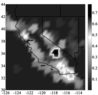Figure 1

As an example, Figure 1 shows the spatial distribution of the measure $\mu_{\Delta}$ on April 1, 2010. It is easy to see two zones with large values of $\mu_{\Delta}$. This is the zone in the south of central California near the border with the state of Nevada and the zone on the border with Mexico. Inside the second zone falls the epicenter of the $M = 7.2$ earthquake on April 4, 2010, located in the north of Mexico. Slightly smaller values of $\mu_{\Delta}$ are fixed in two zones: the coast of central California and the ocean near the coast in northern California. In April 2010, earthquakes with $M = 4.0 - 4.5$ occurred in these zones.

Within the considered period 258 seismic events with $M \geq 5$ occurred in the studied area including the aftershocks and 146 main shocks. A significant part of them occurred with the activity measure $\mu_{\Delta}$ exceeding the level of 0.45.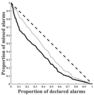Figure 2

We evaluated the effectiveness of the method using an error diagram [Molchan, 1991; Shebalin, 2006]. The considered space and time are divided into cells of 1 month$\:\times\: 0.5$°. If we introduce the threshold $\mu_0$ for the measure $\mu_{\Delta}$, then exceeding this threshold can be considered as a prediction of an earthquake with $M \geq 5$ in a given spatial cell during the subsequent time interval. We specified earthquakes with $M \geq 5$ with epicenters outside the cells with prediction as missed targets. Their proportion is shown in the error diagram along the ordinate axis (Figure 2). The abscissa shows the probability of an accidental earthquake $M \geq 5$ in a cell with a prediction in accordance with a given model of seismicity [Shebalin et al., 2014]. As a rule, the simplest model of seismicity is used, in which the probability of an earthquake is proportional to the number of earthquakes registered in a given cell, with a magnitude, for example, $M \geq 4$. Therefore, the probability of accidental entry is often interpreted as the proportion of space-time of alarms weighted by seismicity [Kossobokov and Shebalin, 2003]. The greater the deviation of the error diagram from the diagonal (the diagonal corresponds to random guessing), the more effective the algorithm is [Shebalin, 2006]. It can be seen from the diagram that the effectiveness of the developed method of monitoring the level of the seismic regime is considerably high. The results obtained are significantly different from random guessing.

#### Kamchatka

The SARD approach has been implemented for the seismically active region of the Kamchatka Peninsula. The catalog of earthquakes of Kamchatka and the Commander Islands (Kamchatka branch of the Geophysical Service of Russian Academy of Sciences. Earthquake catalog of the Kamchatka Peninsula and the Commander Islands, http://www.emsd.ru/sdis/earthquake/catalogue/ catalogue.php)

for the period 1962–2015 was used with a depth of hypocenters not exceeding 70 km [Fedotov and Solomatin, 2015; Levina et al., 2013]. The catalog contains earthquakes with $M_{\mathrm{L}} \geq 3.5$, where $M_{\mathrm{L}}$ is the Kamchatka regional magnitude [Abubakirov et al., 2018]. We used the following values for the input parameters: $q = -2.5$, time period $t_i$ – 1 month, latitude-longitude grid spacing – 0.5°, spacing by $\beta$ – 0.05, $\Delta$ – 12 months, $p = 2$.

The analysis of the results of the monitoring of the level of seismic activity in Kamchatka, obtained with the SARD method, was carried out according to earthquake data since 1980, due to the fact that from that time the catalog is sufficiently complete for the entire studied area.

For the period 1980–2015 in Kamchatka, according to the catalog (Kamchatka branch of the Geophysical Service of Russian Academy of Sciences. Earthquake catalog of the Kamchatka Peninsula and the Commander Islands. (http://www.emsd.ru/sdis/earthquake/catalogue/ catalogue.php),

there were 6 earthquakes with $M_{\mathrm{L}} \geq 6.5$ without excluding the aftershocks. Five of them occurred when measure $\mu_\Delta$ exceeded the level of 0.45, three of them occurred with $\mu_\Delta > 0.55$.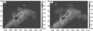Figure 3

Figure 3 provides an example of the spatial distribution of the values of measure $\mu_\Delta$ on March 1, 1992 (Figure 3a) and December 1, 1997 (Figure 3b), white asterisks show epicenters of earthquakes with $M_{\mathrm{L}} \geq 6.5$, that occurred on March 2, 1992 and on December 5, 1997, respectively.

Figure 3 shows the zones with high values of the measure of seismic activity $\mu_\Delta$ along the coast of central Kamchatka. It can be seen in Figure 3a that the epicenter of the earthquake that occurred on March 2, 1992 with $M_{\mathrm{L}} = 6.6$ ($M_{\mathrm{w}}^{\mathrm{GCMT}} = 6.8)$, is located in the northeast of Avacha Bay in the zone for which the values $\mu_\Delta > 0.64$ were the maximum for the entire region in the figure at the beginning of the corresponding month. The epicenter of the earthquake on December 5, 1997 with $M_{\mathrm{L}} = 7.0$ ($M_{\mathrm{w}}^{\mathrm{GCMT}} = 7.8)$ is located in the southeast of the Kamchatka Gulf in the zone with values $\mu_\Delta > 0.56$ and is shown in Figure 3b with white asterisk.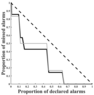Figure 4

We evaluated the effectiveness of the developed method using error diagram [Shebalin et al., 2014] (Figure 4). For this purpose, the considered time and space were divided into cells. The earthquake forecast with $M_{\mathrm{L}} \geq 6.5$ was estimated as exceeding a certain threshold by measure $\mu_\Delta$. Errors such as "missed targets" were the earthquakes with $M_{\mathrm{L}} \geq 6.5$, falling beyond the cells with accurate prediction. In the error diagram (Figure 4), the abscissa shows the probability of an accidental earthquake with $M_{\mathrm{L}} \geq 6.5$ in the cell with a prediction, the ordinate shows the fraction of errors such as "missed targets", the diagonal corresponds to random guessing [Dzeboev, 2017; Shebalin et al., 2011]. To estimate an accidental entry of a strong earthquake in a cell with a prediction, the simplest model of seismicity was used in which the probability of an earthquake is proportional to the number of earthquakes with $M_{\mathrm{L}} \geq 5.5$ recorded in a given spatial cell [Kossobokov and Shebalin, 2003; Shebalin et al., 2014]. The greater the deviation of the error diagram from the diagonal, the more effective the algorithm is [Shebalin et al., 2011]. Figure 4 shows that the proposed method is effective. The presented results differ from random guessing.

#### The Caucasus

We use the SARD method for the territory of the Caucasus within 40–44° N and 41–51° E. The catalog of earthquakes was compiled using the catalogs "Earthquakes in the USSR" (1962–1991) [Nauka, 1997] and "Earthquakes of Northern Eurasia" (1992–2008) [GS RAN, 2013] with depths of hypocenters not exceeding 70 km. To monitor the level of activity of the seismic process, we chose the epicenters of earthquakes with $M \geq 3.0$. It is worth noting that in connection with the absence of a homogeneous catalog of magnitudes for the considered region. The known correlation relationships between magnitudes were applied

[Bormann, 2012; Rautian et al., 2007]. We used the following values for the input parameters: $q = -3.0$, time period $t_i$ – 1 month, latitude-longitude grid spacing – 0.5°, spacing by $\beta$ – 0.05, $\Delta$ – 12 months, $p = 2$.

Analysis of the results of the monitoring of the level of seismic activity in the Caucasus obtained with the help of the SARD method has been carried out based on earthquake data since 1990. From that time the catalog is sufficiently complete for the entire studied area.

In 1990–2008, in the Caucasus, according to the compiled catalog, more than 100 earthquakes with $M \geq 5.0$ occurred excluding the aftershocks. Most of them occurred at the measure $\mu_\Delta$ exceeding the level of 0.5.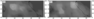Figure 5

Figure 5 provides an example of the spatial distribution of the values of measure $\mu_\Delta$ on December 1, 1994 (Figure 3a) and September 1, 1999 (Figure 3b), white asterisks show epicenters of earthquakes with $M > 5.0$, that occurred on December 12, 1994 and September 19, 1999, respectively.

In Figure 5a the earthquake epicenter with $M = 5.1$ was located in the area, for which the values of $\mu_\Delta > 0.55$ were the maximum for the entire region in the figure at the beginning of the corresponding month. The epicenter of the earthquake in Figure 5b with $M = 5.4$ is shown with white asterisk, located within the area with the values of $\mu_\Delta > 0.65$.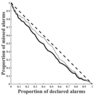Figure 6

To evaluate the effectiveness of the SARD method we constructed an error diagram [Shebalin et al., 2011] (Figure 6). The earthquake forecast with $M \geq 5.0$ was estimated as exceeding a certain threshold by measure $\mu_\Delta$. Errors such as "missed targets" were the earthquakes with $M \geq 5.0$, falling beyond the cells with accurate prediction. To estimate an accidental entry of a strong earthquake in a cell with a prediction, the epicenters of the earthquakes with $M \geq 4.0$ were used. Figure 6 shows that the proposed method is effective. The presented results differ from random guessing.

It is worth noting that the results of applying the SARD method for the territory of the Caucasus are less representative than for Kamchatka and even more so for California [Dzeboev, 2017]. One of the possible reasons may be the known heterogeneity of the catalog.

### Conclusions

• A new mathematical method SARD is proposed for monitoring the level of the seismic regime for estimating the probability of a strong earthquake.
• The possibility of using SARD for the territory of California, the Kamchatka Peninsula and the Caucasus is shown.
• To evaluate the effectiveness of SARD we constructed an error diagram which showed that the proposed method was effective. The obtained results differ from random guessing.

#### Acknowledgment

This work was performed as a part of the grant of the President of the Russian Federation for a state support of young Russian scientists (project number MK-4555.2016.5). The authors are grateful to A. A. Skorkina for discussions and valuable comments, as well as to our colleagues at GC RAS S. M. Agayan, Sh. M. Bogoutdinov and I. O. Belov for their help with the project.

### References

Abubakirov, I. R., A. A. Gusev, E. M. Guseva, V. M. Pavlov, A. A. Skorkina (2018), Mass determination of moment magnitudes $M_w$ and establishing the relationship between $M_w$ and $M_L$ for moderate and small Kamchatka earthquakes, Izvestiya, Physics of the Solid Earth, 54, no. 1, p. 33–47, https://doi.org/10.1134/S1069351318010019.

Agayan, S. M., A. A. Soloviev (2004), Allocation of dense areas in metric spaces basing on crystallization, System Research and Information Technologies, no. 2, p. 7–23.

Agayan, S. M., S. R. Bogoutdinov, M. N. Dobrovolsky (2014), Discrete perfect sets and their application in cluster analysis, Cybernetics and Systems Analysis, 50, no. 2, p. 176–190.

Bormann, P. (ed.) (2012), New Manual of Seismological Observatory Practice 2 (NMSOP-2), Deutsches GeoForschungsZentrum, Potsdam.

Dzeboev, B. A. (2017), A New Approach to Monitoring Seismic Activity: California Case Study, Doklady Earth Sciences, 473, Part 1, p. 338–341, https://doi.org/10.1134/S1028334X17030126.

Fedotov, S. A., A. V. Solomatin (2015), The long-term earthquake forecast for the Kuril-Kamchatka island arc for the September 2013 to August 2018 period; the seismicity of the arc during preceding deep-focus earthquakes in the sea of Okhotsk (in 2008, 2012, and 2013 at $M = 7.7$, 7.7, and 8.3), Journal of Volcanology and Seismology, 9, no. 2, p. 65–80, https://doi.org/10.1134/S0742046315020025.

GS RAN, (publ.) (2013), Earthquakes in the Northern Eurasia in 1992–2008, Catalogue, GS RAN, Moscow, OIFZ RAN, 1997 – Obninsk, 1997-2013, Obninsk.

Gvishiani, A., J. Dubois (2002), Artificial intelligence and dynamic systems for geophysical applications, 350 pp., Springer-Verlag, Paris.

Gvishiani, A. D., B. A. Dzeboev (2015), Assessment of seismic hazard in choosing of a radioactive waste disposal location, Mining Journal, no. 10, p. 39–43, https://doi.org/10.17580/gzh.2015.10.07 (in Russian).

Gvishiani, A., et al. (1994), Identification of a geological region for earthquakes using syntactic pattern recognition of seismograms, Natural Hazards, 10, p. 139–147.

Gvishiani, A. D., S. M. Agayan, Sh. R. Bogoutdinov, A. V. Ledenev, Z. Zlotniki, Z. Bonnin (2003), Mathematical Methods of Geoinformatics. II. Fuzzy-Logic Algorithms in the Problems of Abnormality Separation in Time Series, Cybernetics and Systems Analysis, 39, no. 4, p. 555–563, https://doi.org/10.1023/B:CASA.0000003505.56410.4f.

Gvishiani, A. D., S. M. Agayan, Sh. R. Bogoutdinov, S. Tikhotsky, J. Hinderer, J. Bonnin, M. Diament (2004), Algorithm FLARS and recognition of time series anomalies, System Research &amp; Information Technologies, no. 3, p. 7–16.

Gvishiani, A. D., S. M. Agayan, Sh. R. Bogoutdinov (2008a), Discrete mathematical analysis and monitoring of volcanoes, Inzh. Ekol., no. 5, p. 26–31.

Gvishiani, A. D., S. M. Agayan, Sh. R. Bogoutdinov (2008b), Fuzzy recognition of anomalies in time series, Doklady Earth Sciences, 421, no. 1, p. 838–842, https://doi.org/10.1134/S1028334X08050292.

Gvishiani, A. D., S. M. Agayan, Sh. R. Bogoutdinov, J. Zlotnicki, J. Bonnin (2008c), Mathematical methods of geoinformatics. III. Fuzzy comparisons and recognition of anomalies in time series, Cybernetics and Systems Analysis, 44, no. 3, p. 309–323, https://doi.org/10.1007/s10559-008-9009-9.

Gvishiani, A. D., S. V. Belov, S. M. Agayan, M. V. Rodkin, V. N. Morozov, V. N. Tatarinov, Sh. R. Bogoutdinov (2008d), Geoinformation technologies: artificial intelligence methods in the assessment of tectonic stability of Nizhnekanskii Massif, Inzh. Ekol., no. 2, p. 3–14.

Gvishiani, A. D., M. N. Dobrovolsky, S. M. Agayan, B. A. Dzeboev (2013a), Fuzzy-based clustering of epicenters and strong earthquake-prone areas, Environmental Engineering and Management Journal, 12, no. 1, p. 1–10.

Gvishiani, A., B. Dzeboev, S. Agayan (2013b), A new approach to recognition of the earthquake-prone areas in the Caucasus, Izvestiya, Physics of the Solid Earth, 49, no. 6, p. 747–766, https://doi.org/10.1134/S1069351313060049.

Gvishiani, A. D., S. M. Agayan, M. N. Dobrovolsky, B. A. Dzeboev (2013c), Objective classification of the epicenters and recognition of the earthquake-prone areas in California, Geoinformatika, no. 2, p. 44–57.

Gvishiani, A. D., B. A. Dzeboev, S. M. Agayan (2016), FCAZm intelligent recognition system for locating areas prone to strong earthquakes in the Andean and Caucasian mountain belts, Izvestiya. Physics of the Solid Earth, 52, no. 4, p. 461–491, https://doi.org/10.1134/S1069351316040017.

Gvishiani, A. D., S. M. Agayan, B. A. Dzeboev, I. O. Belov (2017a), Recognition of Strong Earthquake-Prone Areas with a Single Learning Class, Doklady Earth Sciences, 474, Part 1, p. 546–551, https://doi.org/10.1134/S1028334X17050038.

Gvishiani, A. D., B. A. Dzeboev, I. O. Belov, N. A. Sergeyeva, E. V. Vavilin (2017b), Successive Recognition of Significant and Strong Earthquake-Prone Areas: The Baikal–Transbaikal Region, Doklady Earth Sciences, 477, Part 2, p. 1488–1493, https://doi.org/10.1134/S1028334X1712025X.

Gvishiani, A. D., B. A. Dzeboev, N. A. Sergeyeva, A. I. Rybkina (2017c), Formalized Clustering and the Significant Earthquake-Prone Areas in the Crimean Peninsula and Northwest Caucasus, Izvestiya. Physics of the Solid Earth, 53, no. 3, p. 353–365, https://doi.org/10.1134/S106935131703003X.

Keilis-Borok, V., A. Ismail-Zadeh, V. Kossobokov, P. Shebalin (2001), Non-linear dynamics of the lithosphere and intermediate-term earthquake prediction, Tectonophysics, 338, no. 3–4, p. 247–260.

Kossobokov, V., P. Shebalin (2003), Earthquake Prediction, Nonlinear Dynamics of the Lithosphere and Earthquake Prediction, Keilis-Borok V. I. and Soloviev A. A. (eds.), Springer Series in Synergetics, p. 141–207, Springer, Berlin, Heidelberg, https://doi.org/10.1007/978-3-662-05298-3_4.

Laverov, N. P., A. A. Malovichko, O. E. Starovoit (2008), Russian Network of Seismological Observations: Status and Prospects of Development, Materials of International Conference "Seismicity of Northern Eurasia", July 28–31, 2008, p. 5–16, GS RAS, Obninsk.

Levina, V. I., A. V. Lander, S. V. Mityushkina, A. Yu. Chebrova (2013), The seismicity of the Kamchatka region: 1962–2011, Journal of Volcanology and Seismology, 7, no. 1, p. 37–57, https://doi.org/10.1134/S0742046313010053.

Mikhailov, V. O., A. Galdeano, M. Diament, A. D. Gvishiani, S. M. Agayan, Sh. R. Bogoutdinov, E. M. Graeva, P. Sailhac (2003), Application of artificial intelligence for Euler solutions clustering, Geophysics, 68, no. 1, p. 168–180.

Molchan, G. (1991), Structure of optimal strategies in earthquake prediction, Tectonophysics, 193, no. 4, p. 267–276, https://doi.org/10.1016/0040-1951(91)90336-Q.

Nauka, (publ.) (1997), Earthquakes in USSR in 1962–1991, Catalogue, Nauka, Moscow.

Novikova, O. V., I. M. Rotvain (1996), Advance earthquake prediction by algorithm CN, Doklady Akademii Nauk, 348, no. 4, p. 548–551.

Rautian, T. G., et al. (2007), Origins and methodology of the Russian energy K-class system and its relationship to magnitude scales, Seismological Research Letters, 78, p. 579–590.

Riznichenko, Yu. V. (1967), Seismic activity and energy of maximal earthquakes, Problems on Geophysics of Central Asia and Kazakhstan, p. 36–51, Nauka, Moscow.

Shebalin, P. N. (2006), A Methodology for Prediction of Large Earthquakes with Waiting Times Less than One Year, Vychislitel'naya Seismologiya, no. 37, p. 7–182.

Shebalin, P., et al. (2011), Short-term earthquake forecasting using early aftershock statistics, Bulletin of the Seismological Society of America, 101, no. 1, p. 297–312.

Shebalin, P., C. Narteau, M. Holschneider, J. Zechar (2014), Combining earthquake forecast models using differential probability gains, Earth, Planets and Space, 66, no. 37, p. 1–14.

Soloviev, An., et al. (2012a), Automated recognition of spikes in 1 Hz data recorded at the Easter Island magnetic observatory, Earth Planets Space, 64, no. 9, p. 743–752, https://doi.org/10.5047/eps.2012.03.004.

Soloviev, An. A., S. M. Agayan, A. D. Gvishiani, Sh. R. Bogoutdinov, A. Chulliat (2012b), Recognition of disturbances with specified morphology in time series: Part 2. Spikes on 1-s magnetograms, Izvestiya, Physics of the Solid Earth, 48, no. 5, p. 395–409, https://doi.org/10.1134/S106935131204009X.

Soloviev, A., et al. (2013), Mathematical Tools for Geomagnetic Data Monitoring and the INTERMAGNET Russian Segment, Data Science Journal, 12, p. WDS114–WDS119, https://doi.org/10.2481/dsj.WDS-019.

Soloviev, A. A., A. D. Gvishiani, A. I. Gorshkov, M. N. Dobrovolsky, O. V. Novikova (2014), Recognition of earthquake-prone areas: Methodology and analysis of the results, Izvestiya, Physics of the Solid Earth, 50, no. 2, p. 151–168, https://doi.org/10.1134/S1069351314020116.

Soloviev, A. A., J. I. Zharkikh, R. I. Krasnoperov, B. P. Nikolov, S. M. Agayan (2016), GIS-oriented solutions for advanced clustering analysis of geoscience data using ArcGIS platform, Russian Journal of Earth Sciences, 16, p. ES6004, https://doi.org/10.2205/2016ES000587.

Received 22 May 2018; accepted 5 June 2018; published 27 June 2018.Citation: Dzeboev B. A., R. I. Krasnoperov (2018), On the monitoring of seismic activity using the algorithms of discrete mathematical analysis, Russ. J. Earth Sci., 18, ES3003, doi:10.2205/2018ES000623.

Generated from LaTeX source by ELXpaper, v.1.5 software package.